Importance: Medium ✭✭
 Subject: Number Theory » Combinatorial Number Theory
 Keywords: subsequence sum zero sum
Conjecture   For every, the sequence in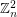consisting of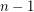copes of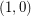andcopies ofhas the fewest number of distinct subsequence sums over all zero-free sequences fromof length.
Definition: Given a sequenceof elements from an additive abelian group, we call a subsequence sum any group element expressable as a sum of some nontrivial subsequence of. We say thatis zero-free ifis not a subsequence sum.
It is easy to see that every sequence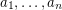of elements from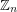has a nontrivial subsequence which sums to zero (actually this holds for every group of order). Just consider the elements,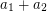,,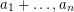. If these elements are distinct, we have a zero sum. Otherwise, we havefor some, but then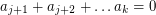. The same argument shows that whenever, every zero-free sequence ofelements ofmust have at leastdistinct subsequence sums. In other words, the sequence consisting ofcopies ofhas the fewest number of distinct subsequence sums over all zero-free sequences inof length.
In the group, a theorem of Olsen shows that every sequence of lengthhas a nontrivial subsequence which sums to zero. However, we do not know what the minimum number of distinct subsequence sums is for a zero-free sequence of a given length. The above conjecture would appear to be the natural optimum.